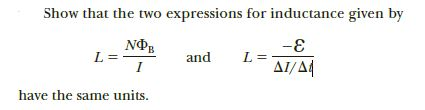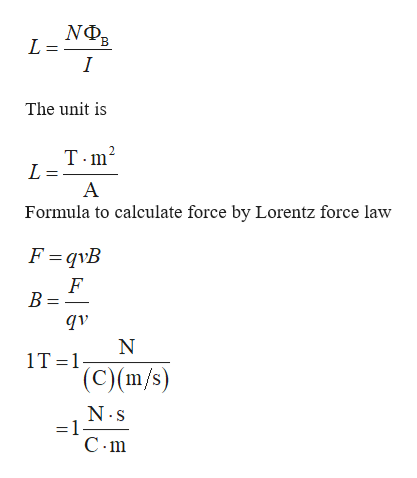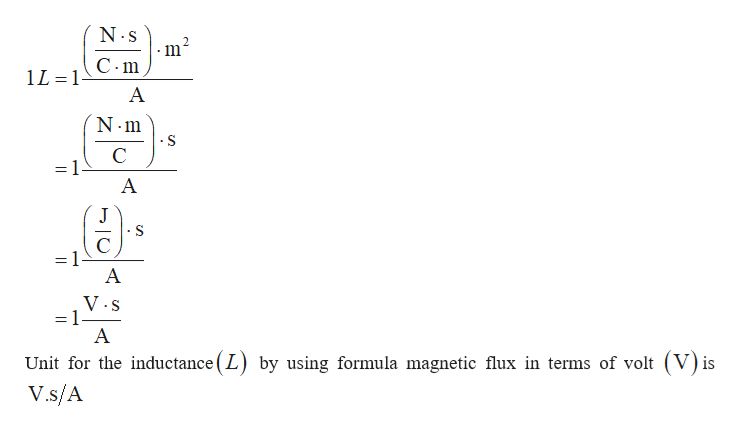# Show that the two expressions for inductance given byNФL =-EandAI/A4have the same units.

Question
1 viewshelp_outlineImage TranscriptioncloseShow that the two expressions for inductance given by NФ L = -E and AI/A4 have the same units. fullscreen
check_circle

Step 1

Formula to calculate the inductance  in terms of magnetic flux ishelp_outlineImage TranscriptioncloseNOB The unit is T.m? L = Formula to calculate force by Lorentz force law F=qvB B = лb 1T=1 (C)(m/s) - N.s =1- C- m || fullscreen
Step 2

The unit of inducta...help_outlineImage TranscriptioncloseN.s C-m 1L =1 A -S V.S A Unit for the inductance ( L) by using formula magnetic flux in terms of volt (V) is V.s/A || fullscreen

### Want to see the full answer?

See Solution

#### Want to see this answer and more?

Solutions are written by subject experts who are available 24/7. Questions are typically answered within 1 hour.*

See Solution
*Response times may vary by subject and question.
Tagged in

### Physics